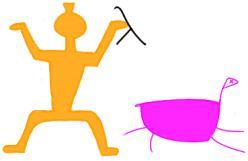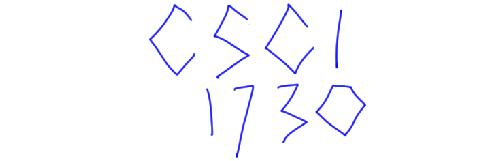### Continuations

You must complete this assignment with a new partner. This must be your second distinct partner for the course. You and your partner must each understand the answers to both problems, so don't just do one each.

### Problem 1

Any program that consumes some amount of stack, when converted to CPS and run, suddenly consumes no stack space at all. Why?

Does this mean that every program should be converted to CPS? Why or why not?

### Problem 2

CPS the following Scheme function. You don't need to CPS primitives such as `empty?`, `first`, `rest`, `cons`, `cons?` and `<`. You may also assume that the function argument to both of the functions is in CPS. Name the result `filter/k` as shown below.

```;; filter: (x -> bool) (listof x) -> (listof x)
(define (filter f l)
(cond
[(empty? l) empty]
[else (cond
[(f (first l)) (cons (first l)
(filter f (rest l)))]
[else (filter f (rest l))])]))

;; filter/k: (x receiver -> doesn't return) (listof x) receiver -> doesn't return
(define (filter/k f l k)
'fill-me-in)
```

Now change the following expressions to use `filter/k`.

```(define (less-than-three x)
(< x 3))
(filter less-than-three
(cons 1 (cons 4 empty))) ;; this evaluates to (list 1)

```

### Problem 3

The Java security model uses a primitive called stack inspection. Let us implement a simple version of this. Assume the language had two new primitives:

```<E> ::= ...
| {bless <E>}
| {check}
```

The `bless` primitive creates a blessed stack frame for the duration of evaluating its sub-expression. The `check` primitive traverses the run-time stack; if it finds a blessed frame it evaluates to `0` (so you can, for instance, use it inside sums without affecting the outcome), otherwise it terminates the program's execution.

Extend the CPSed interpreter presented in the text in Figure 20.2 with these two new language constructs. For your convenience, here is a file to start with: stackinspect.ss

Note: Java lacks tail-call optimization ostensibly because this hurts the ability to implement stack inspection. In fact, this claim is entirely false. But we're stuck with the result of this decision, making the JVM a poor target for other language compilers. Unfortunately, .NET has also adopted this mistake, as the paper explains.

### Handin

Turn in four files:

1. `whynostack.txt`, which contains your solution to Problem 1.
2. `filterk.ss`, which contains your solution to Problem 2.
3. `stackinspect.ss`, which contains your solution to Problem 3.
4. A README file containing the names of the members of your group.